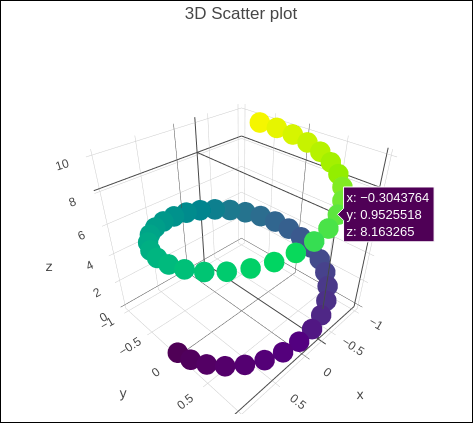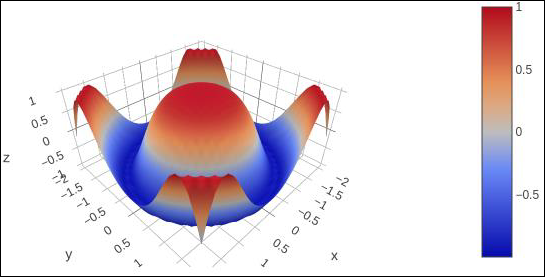# Plotly - 3D Scatter and Surface Plot

This chapter will give information about the three-dimensional (3D) Scatter Plot and 3D Surface Plot and how to make them with the help of Plotly.

## 3D Scatter Plot

A three-dimensional (3D) scatter plot is like a scatter plot, but with three variables - x, y, and z or f(x, y) are real numbers. The graph can be represented as dots in a three-dimensional Cartesian coordinate system. It is typically drawn on a two-dimensional page or screen using perspective methods (isometric or perspective), so that one of the dimensions appears to be coming out of the page.

3D scatter plots are used to plot data points on three axes in an attempt to show the relationship between three variables. Each row in the data table is represented by a marker whose position depends on its values in the columns set on the X, Y, and Z axes.

A fourth variable can be set to correspond to the color or size of the markers, thus, adding yet another dimension to the plot. The relationship between different variables is called correlation.

A Scatter3D trace is a graph object returned by go.Scatter3D() function. Mandatory arguments to this function are x, y and z each of them is a list or array object.

For example −

import plotly.graph_objs as go
import numpy as np
z = np.linspace(0, 10, 50)
x = np.cos(z)
y = np.sin(z)
trace = go.Scatter3d(
x = x, y = y, z = z,mode = 'markers', marker = dict(
size = 12,
color = z, # set color to an array/list of desired values
colorscale = 'Viridis'
)
)
layout = go.Layout(title = '3D Scatter plot')
fig = go.Figure(data = [trace], layout = layout)
iplot(fig)


The output of the code is given below −## 3D Surface Plot

Surface plots are diagrams of three-dimensional data. In a surface plot, each point is defined by 3 points: its latitude, longitude, and altitude (X, Y and Z). Rather than showing the individual data points, surface plots show a functional relationship between a designated dependent variable (Y), and two independent variables (X and Z). This plot is a companion plot to the contour plot.

Here, is a Python script to render simple surface plot where y array is transpose of x and z is calculated as cos(x2+y2)

import numpy as np
x = np.outer(np.linspace(-2, 2, 30), np.ones(30))
y = x.copy().T # transpose
z = np.cos(x ** 2 + y ** 2)
trace = go.Surface(x = x, y = y, z =z )
data = [trace]
layout = go.Layout(title = '3D Surface plot')
fig = go.Figure(data = data)
iplot(fig)


Below mentioned is the output of the code which is explained above −